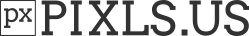# New interesting command 'rbf' to interpolate 1D/2D/3D data.

Hello folks,
I’ve added a new command in the standard library of G’MIC 2.9.1, and I think this could interest some of the G’MIC script writers here. I’m pretty sure this could lead to fun experimentationsRelease of stable G’MIC 2.9.1 is planed for tomorrow.

The new command is named rbf, which stands for Radial Basis Function. More precisely, it implements a RBF-based interpolation of sparse keypoints. To be honest, I’ve already been using RBF-interpolation in some of the G’MIC filters for a long time (commands x_warp, x_morph and clut, to name a few), but I’ve decided it was time to provide this generic interpolation method as a stand-alone command that can be used to interpolate any kind of data.

It basically works like this: the command takes as input a set of K keypoints (X_k,V_k) (where k=1\dots K). The X_k represents the coordinates of keypoint k (X_k can be a 1D/2D or 3D vector), and V_k is the associated value of keypoint k (V_k can be a N-dimensional vector, with N having any value). The set of keypoints is passed to rbf as a single-column (or single-row) vector-valued image, where each pixel represents a keypoint.
From this, the command rbf is able to interpolate the values of the keypoints in all 1D/2D or 3D image space. You specify the size of the interpolated image you want as a result, as well as a corresponding XYZ coordinate mapping (optionally).

To sum up, rbf creates a smooth function that passes through given keypoints.
Beware, if the number of keypoints increases, then processing time explodes. Even if the command is parallelized, it implies a K\times K matrix inversion (K, the number of keypoints), which usually has a complexity of O(K^3) + it has a reconstruction phase with complexity O(whdK). But if the set of keypoints is small enough (I’d say <1000), then it’s a very interesting toy to play with.

A few examples:

• 1D interpolation:
test1d :
20,1,1,2,[u(700),u(150,250)]  # Pick random keypoints
+rbf. 700                     # Interpolate keypoints
700,400,1,3,255 round. graph. ..,1,0,0,400
eval "ellipse(#-1,i0,i1,5,5,0,0.5,255,0,0); I"


test1d gives:

• 2D interpolation:
test2d :
sp duck
100%,100% noise_poissondisk. 10  # Pick random keypoints
1,{is},1,5 eval[-2] "begin(p=0);i?(I[#-1,p++]=[x,y,I(#0)])" # Create set of 2D colored keypoints
to_rgb mul[0,1] dilate_circ 5
+rbf[-1] {0,[w,h]} c[-1] 0,255 rm..


test2d gives (after heating your CPUs a lot…):

You can use rbf to create super-easy random color gradients:
test2d_2 :
32,1,1,5,u([400,400,255,255,255])
rbf 400,400 c 0,255


test2d_2 gives: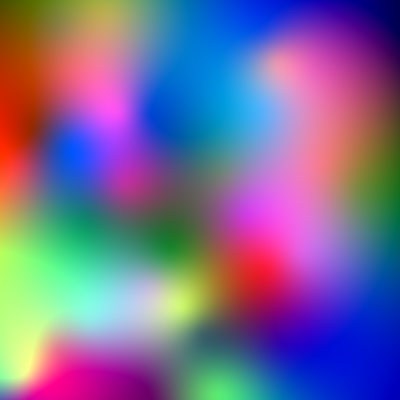That’s it. I’d be interested by what you can obtain with this new command.
Note that we already had solidify and inpaint_pde for doing such interpolation, but having another option is still cool.

Spooky… you must be some kind of mind reader! Was wondering about this exact thing last night. Can’t wait to try some things with it, thanks!

1 Like

Is this in any way related to graph-based processing? I find those papers interesting but don’t know where to start.

Not, that is not directly related in the sense that we never have to build a graph structure from the set of keypoints (e.g. Delaunay triangulation) to interpolate them.

Surprised to find this works reasonably well too:

gcd_bnz : skip ${1=1} # blur non-zero repeat$! l[$>] +eq 0 +*.. . eq.. 0 b$1 -[0,-1] +eq. 0 +[-2,-1] /[0,-1]
endl done


gmic sp duck 100%,100%,1,1 noise_poissondisk. 10 mul +gcd_bnz 6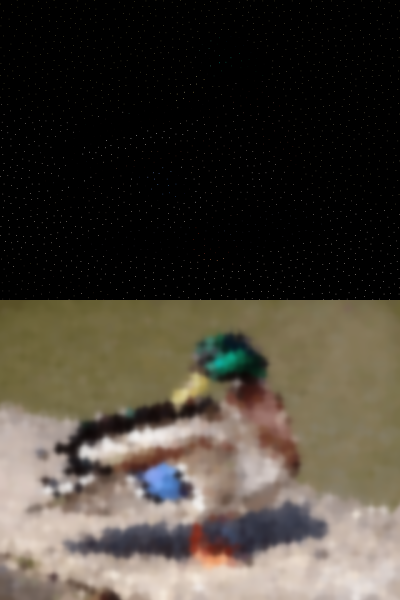Indeed!
But unfortunately, with this method you don’t preserve the color values at the locations of the samples.

Definitely a major drawback compared to rbf and similar. But I do like fast approximatesIt’s a quick way to create gradients at least, also possible to use it as a very basic inpaint by combining with the original.

The use of inpaint_pde can be also considered, as a mix between RBF and your technique, it’s faster than RBF, particularly when the number of keypoints is high (it doesn’t even depend on the number of keypoints), and is based on image smoothing, as with blur.

\$ gmic sp duck 100%,100%,1,1 noise_poissondisk. 10 mul  negate. inpaint_pde.. .


1 Like

Yes, seems easy enough to tweak for performance too. We have a crazy number of inpaint options now for sure!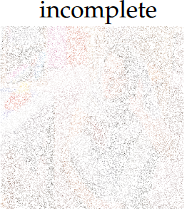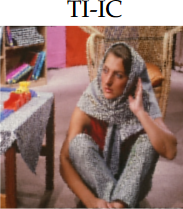1 Like

Interesting, by following links in there it looks like I effectively implemented EFAN algorithm but using a standard gaussian blur instead of sparse. The method I used could in theory work in plain GIMP using layers now that it has floating point images.

The incomplete images they use have higher percentage pixels than we used here (about equivalent to noise_poissondisk 3.5)

Hmm, if it can be done with layers, I suppose one can use multiple gaussian blur filter layers on Krita to do the same, right?

Yes, if it has floating point data I think so. There’s redundant stuff in my example above that I forgot to clean up (for inpainting). It’s just:

1. input image with sparse points, zeros elsewhere
2. new layer from that = set to 1 where input is non-zero (and zero elsewhere)
3. blur both layers (ensure radius is large enough)
4. optional: set any zeros in second layer to 1 to avoid div by zero (NaN)
5. divide input layer by the second

Edited for mistake in step 2…

Edit2: I tested that in GIMP and it’s fine. The second layer needs to be max value (e.g. 255 for sRGB) instead of 1 and then it works.